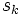# Finite abelian groups with the same order statistics are isomorphic

## Statement

Suppose$G$ and$H$ are Finite abelian group (?)s that are order statistics-equivalent: the order statistics of$G$ equal the order statistics of$H$. Then,$G$ is isomorphic to$H$.

## Facts used

1. Structure theorem for finite abelian groups

## Proof

### Proof idea

We show that the invariants needed to describe a finite abelian group by the structure theorem are completely determined by the order statistics.

### Proof details

By fact (1), any finite abelian group can be written as a direct product of cyclic groups of prime power order, with the number of copies of a cyclic group of prime power order independent of the choice of decomposition.

We claim that the order statistics of a finite group determine the number of times each cyclic group of prime power order occurs as a direct factor. First, for every prime$p$, consider the subgroup of elements whose order is a power of$p$ (in other words, the$p$-Sylow subgroup). This is obtained by grouping together, in the direct product decomposition, all cyclic groups of order a power of$p$. Thus, we may restrict attention to this subgroup, so it suffices to consider the case that$G$ is a finite abelian$p$-group.

For any$k$, let$s_k$ be the number of cyclic group factors of order$p^k$, let$s$ be the sum of the$s_k$s, and let$t_k$ be the logarithm to base$p$ of the number of elements of order dividing$p^k$. This makes sense since theelements of order dividing$p^k$ form a subgroup. Also,$t_k$ is clearly determined by the order statistics. We claim that the$t_k$s determine the$s_k$s.

An easy count shows that for all$k$:$t_k = \sum_{i < k} is_i + k\sum_{j \ge k} s_j$.

We thus get, taking a first difference:$t_{k+1} - t_k = \sum_{j > k} s_j$.

Taking a second difference yields:$2t_k - t_{k+1} - t_{k-1} = s_k$.

This shows that the$t_k$s determine the$s_k$s, completing the proof.